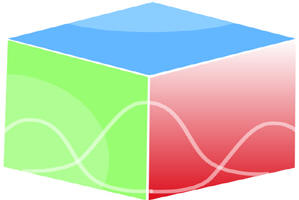``Home| Journals | Statistical Calculator | About Us | Contact Us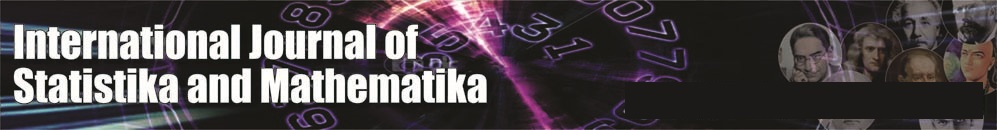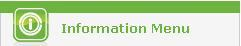Untitled Document

On Strongly l(m, n) - J - continuous functions

D.Sasikala1, I.Arockiarani2

1Department of Mathematics with Computer Applications, Nirmala College for Women, Coimbatore, (TN) INDIA

2Department of Mathematics, Nirmala College for Women,Coimbatore, (TN) INDIA

Research Article

Abstract: In this paper, we introduce the concept of l(m. n) - J- closed sets in bigeneralized topological spaces and study some of their properties. The notion of Jg(m, n)- continuous functions and strongly l(m. n) - J- closed set is also defined on bigeneralized topological spaces and investigate some of their characterizations.

Mathematics Subject Classification: 54A05, 54A10

Keywords:  bigeneralized topological spaces, l(m.n)-J-closed sets,        Jg(m,n)- continuous           functions, l(m, n) – J s – closed set.

1. Introduction

Á.Császár  introduced the concepts of generalized neighborhood systems and he also introduced the concepts of continuous functions and associated interior and closure operators on generalized neighborhood systems and generalized topological spaces. In particular, he investigated characterizations for generalized continuous functions (= (g,g)- continuous functions).  In , he introduced and studied the notions of g- a - open sets, g - semi-open sets,  g – pre open sets and g - b open sets in generalized topological spaces.

After the introduction of the concept of generalized closed set by Levine in a topological spaces. Several other authors gave their ideas to the generalizations of various concepts in topology. Kelly introduced the concept of bitopological spaces .Since many mathematicians generalized the topological concepts into bitopological setting. In 1986, Fukutake  generalized the notion to bitopological spaces and he defined a set ij-generalized closed set. Some new types of generalized closed sets in bitopological spaces were introduced. In this paper, we introduce the notion of lm, n- J - closed sets in bigeneralized topological spaces and study some of their properties. We also introduce the concept of strongly lm, n- J - closed sets  and strongly lm, n- J -continuous functions and investigate some of their characterizations.

2. Preliminaries

Let X be a non – empty set and l be a collection of subsets of X. Then l is called a generalized topology (briefly GT) on X iff  f Î l and Gi Î l for i Î I ¹ f implies G =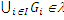.We call the pair (X,l), a generalized topological space(briefly GTS)on X. The elements of l are called l- open sets and the complements are called l- closed sets. The generalized closure of a subset S of X, denoted by cl(S), is the intersection of generalized closed sets including S and the interior of S, denoted by il(S), is the union of generalized open sets contained in S.

Definitions 2.1. Let (X, l) be a generalized topological space and A Í X, then A is said to be

(1)   l - semi open if A Í cl (il (A))

(2)   l - pre open if A Í il( cl(A))

(3)   l - a- open if A Í il( cl (il (A)))

(4)   l - b open if A Í cl (il (cl(A)))

The complement of  l - semi open (resp  l - pre open , l - a- open, l - b open) is said to be l - semi closed (resp  l - pre closed , l - a- closed, l - b closed). The class of all l - semi open sets on X is denoted by s(lx)(briefly s or s). The class of l - pre open (l - a- open and  l - b open) sets on X as [p(gx), a(gx), b(gx) ] or briefly [[p, a, b].

Definition 2.3.  Let X be non-empty set and let l1 and l2 be generalized topologies on X. A triple (X, l1, l2) is said to be a bigeneralized topological space (briefly BGTS).

Let (X, l1, l2) be a bigeneralized topological space and A be a subset of X. The closure of A and the interior of A with respect to lm are denoted by clm (A) and ilm (A), respectively, for m = 1,2.

Definition 2.4. Let (X, l) be a generalized topological space and A Í X, then A is said to be  l - J- open if A Í il (cπ (A)). The complement of  l - J- open is said to be  l - J- closed.

The class of all l - J - open sets on X is denoted by λ – JO(X). The class of all l - J - closed sets on X is denoted by λ – JC(X).The l - J- closure of a subset S of X, denoted by cJ(S) is the intersection of l - J- closed sets including S. The l - J- interior of a subset S of X, denoted by iJ (S) is the union of  l - J- open sets contained in S.

The class of all l - J - open sets is properly placed between l - open sets and l - pre – open sets.

3.   l(m, n) - J- closed sets

Definition 3.1.  A Subset A of a bigeneralized topological space (X, l1, l2) is said to be l(m, n) – J - closed set if cln (A) Í U, whenever A Í U and U is lm – J- open, where m, n = 1,2 and m¹n. The complement of l(m, n) – J - closed set is said to be l(m, n) – J-open set.

Remark 3.2.  Every (m, n) - closed set is l(m, n) – J - closed.

The converse is not true as can be seen from the following example.

Example 3.3. Let X = {a, b, c}.  Consider two generalized topologies l1 = {f,{a},{a, b}, X}, and  l2 = {f,{c},{b, c}},.Let A = {a, b}, then A is l(m, n) – J- closed but not  (m, n) - closed.

Proposition 3.4. Every  l(m, n) – closed set is  l(m, n) – J- closed.

Proof: Let U be a lm – J- open set such that A Í U. Since A is l(m, n) – closed set, cln (A) Í U. Therefore A is l(m, n) – J- closed.

Proposition 3.5.  Let (X, l1, l2) be a bigeneralized topological space and A be a subset of X. If A is ln – closed, then A is l(m, n) – J- closed, where m, n = 1, 2 and m ¹ n.

Remark 3.6.(i)  The union of two l(m, n) – J - closed need not be l(m, n) – J- closed.

(ii) The intersection of two l(m, n) – J- closed sets need not be   l(m, n) – J – closed.

Proposition 3.7.  Let (X, l1, l2) be a bigeneralized topological space. If A is l(m, n) – J - closed and F is (m, n) – J- closed, then A Ç F is l(m, n) – J – closed, where m, n = 1, 2 and m ¹ n.

Proof:  Let U be a lm – J- open set such that A Ç F Í U. Then A  Í U È (X - F) and so       cln (A) Í U È (X - F).Therefore cln (A)  Ç F Í U .Since F is (m, n) – J- closed,  c ln (A Ç F)  Í c ln (A)  Ç  c ln (F) Í U . Hence A Ç F is l(m, n) – J – closed.

Proposition 3.8.  For each element x of a bigeneralized topological space (X, l1, l2), {x} is   lm - J- closed or X – {x} is l(m, n) - J - closed, where m, n = 1, 2 and m ¹ n.

Proof:  Let x Î X and the singleton {x} be not lm – J- closed. Then X – {x} is not lm –J- open, if X Î lm, then X is only lm – J- open set which contains X – {x}, hence X – {x} is l(m, n) – J- closed and if X Ï lm, then X – {x} is l(m, n) – J– closed.

Proposition 3.9.  Let (X, l1, l2) be a bigeneralized topological space. Let A Í X be a l(m, n) – J - closed subset of X, then cln (A) \ A does not contain any non- empty l- J- closed set, where m, n = 1, 2 and m ¹ n.

Proof:  Let A be a l(m, n) – J - closed set and F ¹ f is lm – J- closed such that F Í c ln (A) \ A. Then F Í X \ A and hence A Í X \ F. Since A is l(m, n) – J- closed, c ln (A) Í X \ F and hence F Í X \ c ln (A) .So, F Í cln (A) Ç (X \ cln (A)) = f.Therefore c ln (A) \ A does not contain any non- empty lm   - J- closed set.

Proposition 3.10.  Let l1 and  l2  be generalized topologies on X. If A is l(m, n) - J- closed set, then clm ({x}) Ç A ¹ f holds for each x Î  cln (A), where m, n = 1, 2 and m ¹ n.

Proof:  Let x Î  cln (A). Suppose that clm ({x}) Ç A = f. Then A Í X - clm ({x}). Since A is    l(m, n) – J– closed and X - clm ({x}) is lm – J- open.  Thus cln (A) Í X - clm ({x}). Hence cln (A) Ç  clm ({x}) = f. This is a contradiction.

Proposition 3.11.  If A is a l(m, n) – J - closed set of (X, l1, l2) such that A Í B Í  cln (A) then B is l(m, n) – J - closed set, where m, n = 1, 2 and m ¹ n.

Proof: Let A be a l(m, n) – J- closed set and A Í B Í  cln (A). Let B Í U and U is lm – J-open. Then A Í U. Since A is l(m, n) – J – closed, we have cln (A) Í U. Since B Í  cln (A), then cln (B)  Í cln (A) Í U. Hence B is l(m, n) – J – closed.

Remark.3.12.  l(1,2) – JC(X) is generally not equal to l(2,1) – JC(X) as can be seen from the following example.

Example 3.13.  Let X = {a, b, c}. Consider two generalized topologies  l1 = {f, {a}, {a, b}, X} and l2 = {f, {c}, {b, c}}.Then l(1,2) – JC(X) = {{a}, {b},{a, b}, {b, c},X} and l(2,1) – JC(X) = {{b}, {c},{a, b}, {b, c},f, X }. Thus  l(1,2) – JC(X)  ¹  l(2,1) – JC(X).

Proposition 3.14.  Let l1 and l2 be generalized topologies on X. if l1  Í  l2, then  l(2,1) – JC(X) Í  l(1,2) – JC(X)

Proposition 3.15.  For a bigeneralized topological space (X, l1, l2), lm   - JO(X) Í  ln   - JC(X)

if  and only if every subset of X is  l(m, n) - J - closed set, where m, n = 1, 2 and m ¹ n.

Proof: Suppose that lm   - JO(X) Í  ln   - JC(X). Let A be a subset of X such that A Í U, where U Î  lm   - JO(X). Then cln (U)  Í cln (A) = U and hence A is l(m, n) – J – closed set.

Conversely, suppose that every subset of X is l(m, n) – J – closed. Let U Î  lm   - JO(X).Since U is l(m, n) – J – closed, we have cln (U) Í U. Therefore, U Î ln   - JC(X) and hence lm   - jO(X) Í  ln   - JC(X).

Proposition 3.16.  Let (X, l1, l2) be a bigeneralized topological space. Let A Í X be a l(m, n) – J - closed subset of (X, l1, l2). Then A is l- J- closed if and only if cln (A) \ A is l- J- closed, where m, n = 1, 2 and m ¹ n.

Proof:  Let A be a l(m, n) – J- closed set. If A is lm – J- closed, then cln (A) \ A = f, but f is  lm – J- closed . Therefore c ln (A) \ A is l- J- closed.

Conversely, Suppose that c ln (A) \ A is lm   - J- closed, As A is l(m, n) – J– closed, cln (A) \ A = f, Consequently cln (A) =  A.

Proposition 3.17.  Let (X, l1, l2) be a bigeneralized topological space. If A is l(m, n) – J - closed and A Í B Í  cln (A), then cln (B) \ B has no non empty l- J- closed subset.

Proof: A Í B implies X – B Í X – A and B Í cln (B) implies cln ((cln (A)) = cln (A).Thus cln (B) Ç (X - B) Í cln (A) Ç (X -A) which yields cln (B) / B Í cln (A) / A. As A is l(m, n) – J – closed,    c ln (A) \ A has no non empty lm – J- closed subset. Therefore c ln (B) \ B has no non empty l- J- closed subset.

Remark 3.18.(i)  The intersection of two l(m, n) – J- open sets need not be l(m, n) – J- open

(ii)  The union of two l(m, n) – J- open sets need not be   l(m, n) – J – open.

Proposition 3.19.   A subset of a bigeneralized topological space (X, l1, l2) is l(m, n) - J - open iff every subset of F of X, F Í  iln (A) whenever F is lm – J- closed and F Í A, where m, n = 1, 2 and m ¹ n.

Proof:  Let A be a lm, n – J- open. Let F Í A and F is lm – J-closed. Then X – A Í X – F and X – F is lm – J- open, we have X – A is l(m, n) – J– closed, then cln (X - A)  Í X - F. Thus X - iln (A) Í X – F and hence F Í  iln (A).

Conversely, let F Í  iln (A) where F is a lm – J- closed set such that F Í A. Let X - A Í U where U is a lm – J- open. Then X - U Í A and X - U is lm – J- closed. By the assumption,  X - U Í   iln (A), then X - iln (A)  Í U. Therefore, cln (X - A) Í U. Hence X - A is l(m, n) - J - closed and hence A is l(m, n) -J - open.

Proposition 3.20.  Let A and B be subsets of a bigeneralized topological space(X, l1, l2) such that iln (A) Í B Í A. If A is l(m, n) - J - open then B is also l(m, n) - J- open, where m, n = 1, 2 and m ¹ n.

Proof:  Suppose that iln (A) Í B Í A. Let F be a lm – J- closed set such that F Í  B. Since A is l(m, n) - J - open, F  Í  iln. Since ilÍ B, we have iln (iln(A)) Í iln(B). Consequently iln(A) Í iln(B). Hence F Í iln(B). Therefore, B is l(m, n) - J -open.

Proposition 3.21.   If A be a subset of a bigeneralized topological space (X, l1, l2) is l(m  ,n) - J- closed , then cln (A) - A is lm, n – J – open, where m, n = 1, 2 and m ¹ n.

Proof:  Suppose that A is lm, n – J – closed. Let X – (cln (A) - A) Í U and U is lm – J- open. Then X – U Í X – (cln (A) - A) and X – U is lm – J- closed. Thus we have cln (A) - A does not contain non – empty lm – J- closed by Proposition 3.10 . Consequently, X – U = f, then U = X. Therefore, cln (X- ( cln (A) – A)) Í U, so we obtain X – (cln (A) – A)  is lm, n – J- closed. Hence  cln (A) - A is lm, n - J - open.

4.   Jg(m, n)- continuous functions

Definition 4.1. Let (X,lX1, lX2) and (Y,lY1, lY2) be generalized topological spaces. A function f: (X,lX1, lX2) ® (Y,lY1, lY2) is said to be (m, n) - J- generalized continuous (briefly Jg(m, n)- continuous) if f-1(F) is l(m, n)- J-closed in X for every ln- closed F of Y, where m, n = 1,2 and m¹ n.

A function f: (X,lX1, lX2) ® (Y,lY1, lY2) is said to be pairwise J-generalized continuous(briefly pairwise Jg-continuous) if f is Jg(1,2)-continuous and  Jg(2,1)-continuous.

Proposition 4.2.  For an injective function f: (X,lX1, lX2) ® (Y,lY1, lY2), the following properties are equivalent:

(1)   f is Jg(m, n)- continuous,

(2)   For each x Î X and for every l n- open set V containing f(x), there exists a l(m, n)-J-open set U containing x such that f(U) Í V:

(3)   f(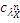(A)) Í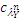(f(A)) for every subset A of X;

(4)(f-1(B)) Í f-1((B) for every subset B of Y.

Proof: (1) Þ(2): Let x Î X and V be a ln-open subset of Y containing f(x). Then by (1),             f -1(V) is l(m, n)-J-open of X containing x. If U = f -1(V), then f (U) Í V.

(2)Þ(3): Let A be a subset of X and f(x) Ï ((f(A)). Then, there exists a l n – open subset V of Y containing f(x) such that V Ç f(A) = f.Then by(2), there exist a l(m, n)-J-open set such that  f(x) Î f(U) Í V. Hence, f(U) Ç f(A) = f implies U Ç A = f. Consequently, x Ï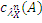and  f(x) Ï f ((A)).

(3) Þ(4): Let B be a subset of Y. By (3) we obtain f((f -1(B)) Í(f(f -1(B)). Thus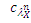(f -1(B)) Í f -1  (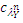(B)).

(4) Þ(1): Let F be a ln – closed subset of Y. Let U be a lm – J- open subset of X such that    f-1 (F) Í U. Since(F) = F and by (4),(f -1(F)) Í U. Hence f is Jg (m, n) continuous.

Definition 4.3.  Let (X,lX1, lX2) ® (Y,lY1, lY2) be generalized topological spaces. A function f: (X,lX1, lX2) ® (Y,lY1, lY2) is said to be Jgm- continuous if f -1(F) is lm –J-closed in X for every lm – closed F of Y, for m = 1,2.

Definition 4.4.  Let (X,lX1, lX2) ® (Y,lY1, lY2) be generalized topological spaces. A function f: (X,lX1, lX2) ® (Y,lY1, lY2) is said to be Jgm- closed(resp. Jgm- open) if f(F) is lm – J-closed(resp lm –J- open) of Y for every lm – closed (resp. lm – open) F of X, for m = 1,2.

Proposition 4.5. If f: (X,lX1, lX2) ® (Y,lY1, lY2) is Jgm - continuous and Jgm - closed , then f(A) is l (m, n)- J-closed subset of Y for every l (m, n)- J-closed subset A of X, where m, n = 1,2 and   m¹ n.

Proof:  Let U be lm – J- open subset of Y such that f (A) Í U. Then A Í f-1(U) and f-1(U) is lm – J-open subset of X. Since A is  l (m, n)- J-closed,(f(A)) Í f-1(U) and hence f((A)) Í U. Therefore we have(f(A)) Í(f((A))) = f((A)) Í U. Therefore, f(A) is l (m, n)- J-closed subset of Y.

Lemma 4.6.  If f: (X,lX1, lX2) ® (Y,lY1, lY2) is Jgm – closed, then for each subset S of Y and each lm – J- open subset U of X containing f -1(S), there exists a lm – J- open subset V of Y such that f-1(V)  Í U.

Proposition 4.7.  If  f: (X,lX1, lX2) ® (Y,lY1, lY2) is injective, Jgm-closed and Jg(m ,n)-continuous, then f-1(B) is l (m, n)- J-closed subset of X for every l (m, n)- J-closed subset of B of Y, where m, n = 1,2 and m¹ n.

Proof:  Let B be a l (m, n)- J-closed subset of Y. Let U be a lm – J- open subset of X such that     f-1(B) Í U. Since f is Jgm-closed and by lemma 4.6, there exists a lm – J- open subset of Y such that B Í V and f-1(V) Í U. Since B is l (m, n)- J-closed set and B Í V, then(B) Í V. Consequently, f-1((B))  Í f-1(V) Í U. By theorem 4.2,(f-1(B)) Í f-1((B) ÍU and hence  f -1(B) is l (m, n)- J-closed subset of X.

Definition 4.8. A bigeneralized topological space (X,lX1, lX2) is said to be l (m, n) – JT1/2 – space if, for every l (m, n)- J-closed set is ln – closed, where m, n = 1,2 and m¹ n.

Definition 4.9. A bigeneralized topological space (X,lX1, lX2) is said to be pairwise l – JT1/2 – space if it is both l (1, 2) –JT1/2 – space and l (2, 1) – J1/2 – space.

Proposition 4.10. A bigeneralized topological space is a  l (m, n)- JT1/2-space if and only if {x} is ln - open or lm –J-closed for each x Î X, where m, n = 1,2 and m¹ n.

Proof: Suppose that {x} is not lm –J- closed. Then X – {x} is l(m, n) -J-closed by proposition 3.9. Since X is l (1, 2) -T1/2 -space, X – {x} is ln -closed. Hence, {x} is ln - open.

Conversely, let F be a l (m, n) - J-closed set. By assumption , {x} is ln - open or lm –J- closed for any x Î cln(F).Case (i) Suppose that {x}is ln - open. Since {x} Ç F¹ f, we have x Î F. Case (ii) Suppose that {x} is lm – J-closed. If x Ï F, then {x} Í cln(F) – F, which is a contradiction to proposition 3.9, Therefore, x Î F. Thus in both case, we conclude that F is ln - closed. Hence, (X,lX1, lX2) is a l (m, n) -JT1/2 -space.

Definition 4.11.  Let (X,lX1, lX2)  and (Y,lY1, lY2) be generalized topological spaces. A function f: (X,lX1, lX2) ® (Y,lY1, lY2) is said to be Jg (m, n) - irresolute if f -1(F) is l(m, n)-J- closed in X for every l(m, n) –J-closed F of Y, where m, n = 1,2 and m¹ n.

Proposition  4.12. Let f: (X,lX1, lX2® (Y,lY1, lY2) and g: (Y,lY1, lY2) ® (Z,lZ1, lZ2) be functions, the following properties hold:

(i)                 If f is Jg (m, n) - irresolute and Jg (m, n) -continuous, then g  °  f  is Jg (m, n) -continuous.

(ii)               If f and g are Jg (m, n) - irresolute , then g  °  f  is Jg (m, n) - irresolute;

(iii)             Let (Y,lY1, lY2) be a l (m, n) -JT1/2 -space. If f and g are Jg (m, n) -continuous, then g ° f is Jg (m, n) -continuous.

Proof:  (i) Let F be a ln -closed subset of Z. Since g is Jg (m, n) - continuous, then g -1(F) is l(m, n) -J-closed subset of Y. Since f is Jg (m, n) - irresolute, then (g °  f)-1(F) = f -1(g -1(F)) is l(m, n) -J-closed subset of X. Hence g °  f  is Jg (m, n) -continuous.

(ii)  Let F be a l(m, n) -J- closed subset of Z. Since g is Jg (m, n) - irresolute, then g -1(F) is l(m, n) -J-closed subset of Y. Since f is Jg (m, n) - irresolute, then (g  °  f)-1(F) = f -1(g -1(F)) is l(m, n) –J- closed subset of X. Hence, g  °  f  is Jg (m, n) - irresolute.

(iii) Let F be a ln -closed subset of Z. Since g is Jg (m, n) -continuous, then g -1(F) is l(m, n) -J- closed subset of Y. Since (Y,lY1, lY2) is a l (m, n) -JT1/2 -space, then g -1(F) is ln -closed subset of Y. Since f is Jg (m, n) -continuous, then (g  °  f)-1(F) = f -1(g -1(F)) is l(m, n) –J-closed subset of X. Hence then g °  f is Jg (m, n) -continuous.

Proposition  4.13. Let: (X,lX1, lX2)  be a l (m, n) -JT1/2 -space. If f: (X,lX1, lX2® (Y,lY1, lY2) is surjective, gn -closed and Jg (m, n) -irresolute, then (Y,lY1, lY2) is a  l (m, n) -JT1/2 -space, where m, n = 1,2 and m¹ n.

Proof:  Let F be a l (m, n) -J-closed subset of Y. Since f is Jg (m, n) - irresolute, we have f  -1(F) is a   l ( m, n) -J-closed subset of X. Since (X,lX1, lX2) is a l (m, n) -JT1/2 -space, f  -1(F) is a  l n -closed subset of X. It follows by assumption that F is a l n -closed subset of Y. Hence (Y,lY1, lY2) is a  l (m, n) -JT1/2 space.

Proposition  4.14. Let: (X,lX1, lX2)  be a l (m, n) - JT1/2 space. If f: (X,lX1, lX2® (Y,lY1, lY2)  is bijective, gn -open and Jg (m, n) – irresolute, then (Y,lY1, lY2) is a  l (m, n) -JT1/2space,  where        m, n = 1,2 and m¹ n.

5. Strongly-λ(m, n) - J - closed sets

Definition 5.1.  A subset A of a bigeneralized topological space (X, l1, l2) is said to be strongly l(m, n) – J s - closed set (briefly l(m, n) – J s - closed) if cln (A) Í U, whenever A Í U and U is    l(m, n) – J - open, where m, n = 1,2 and m¹n.

Definition 5.2.  A subset A of a bigeneralized topological space (X, l1, l2) is said to be pairwise strongly l(m, n) – P- J s closed (briefly l(m, n) – P- J s closed)if A is l(1,2) -Js – closed and l(2,1) - Js – closed..

Proposition 5.3.  Every λn - closed set is l(m, n) – J s – closed.

Proof: Let U be a l(m, n) – J – open set such that A Í U and A be λn - closed set. Then cln (A) = A and so cln (A) Í U. Thus A is l(m, n) – J s – closed.

Proposition 5.4.  Let (X, l1, l2) be a bigeneralized topological space. Let A Í X be a l(m, n) – Js - closed  if and only if , then cln (A) \ A does not contain any non- empty l (m,n)  - J- closed set, where m, n = 1, 2 and m ¹ n.

Proof:  Let F be a l(m, n) – J - closed subset of cln (A) \ A . Now, F Í c ln (A) \ A and  A Í X \ F where A is l(m, n) – J s – closed  and X \ F is l(m, n) – J – open. Thus  c ln (A) Í X \ F and hence F Í X \ c ln (A) .So, F Í cln (A) Ç (X \ cln (A)) = f.Therefore F = j.

Conversely, assume cln (A) \ A contains no non- empty l (m,n)  - J- closed set. Let U be l (m,n)  - J- open such that A Í U. Suppose that cln (A) is not contained in U, then cln (A) Ç Uc  is a nonempty  l (m, n)  - J- closed set of cln (A) \ A, which is a contradiction. Therefore cln (A) Í U and hence A is l(m, n) – J s – closed.

Proposition 5.5.  Let (X, l1, l2) be a bigeneralized topological space. Let A Í X be a l(m, n) – Js - closed  and A Í B Í  cln (A) then B is l(m, n) – Js - closed set, where m, n = 1, 2 and m ¹ n.

Proof: Let A be a l(m, n) – Js- closed set and A Í B Í  cln (A). Let B Í U and U is l(m, n) – J - open. Then A Í U. Since A is l(m, n) – Js – closed, we have cln (A) Í U. Since B Í  cln (A), then cln (B)  Í cln (A) Í U. Hence B is l(m, n) – Js – closed.

Definition 5.6. A subset A of a bigeneralized space X is called strongly l(m, n) – J s – open if Ac is l(m, n) – J s – closed.

Proposition 5.7.  Let (X, l1, l2) be a bigeneralized topological space. Let A Í X be a l(m, n) – Js – open if and only if F Í iln (A) whenever F Í A and F is l(m, n) – J – closed.

Proof: Let A be l(m, n) – Js- open and suppose F Í A where F is a l(m, n) – J – closed. Then X \ A is l(m, n) – J s – closed and X \ A Í X \ F, where X \ F is l(m, n) – J – open set. This implies that  cln (X \ A) Í X \ F. Now cln (X \ A) = X \ iln (A). Hence X \ iln (A) Í X \ F and F Í iln (A).

Conversely. If  F  is an l(m, n) – J – closed with F Í iln (A) whenever F Í A. Then X \ A Í X \F and X \ iln (A) Í X \ F. Thus cln (X \ A) Í X \ F. Hence X \ A is l(m, n) – Js- closed and A is l(m, n) – Js- open.

Proposition 5.8.  Let (X, l1, l2) be a bigeneralized topological space. For each x Î X.{x} is   l(m, n) – J – closed or {x}c is l(m, n) – Js- closed.

Proof: If {x} is not l(m, n) – J – closed, then the only l(m, n) – J – open containing {x}c is X. Thus cln ({x}c) Í X and {x}c is l(m, n) – Js- closed.

6. On Strongly - λ(m, n)  - J - continuous and irresolute functions

Definition 6.1. Let (X,lX1, lX2) and (Y,lY1, lY2) be generalized topological spaces. A function f: (X,lX1, lX2) ®(Y,lY1, lY2) is said to be l(m, n) -strongly J - continuous(briefly λ(m, n)-Js-  continuous) if f-1(F) is l(m, n)- Js-closed in X for every ln- closed F of Y, where m, n = 1,2          and m ¹ n.

Definition 6.2. Let (X,lX1, lX2) and (Y,lY1, lY2) be generalized topological spaces. A function f: (X,lX1, lX2) ®(Y,lY1, lY2) is said to be l(m, n) -strongly J – irresolute (briefly λ(m, n)-Js-  irresolute) if f-1(F) is l(m, n)- Js-closed in X for every l(m, n)- Js-closed F of Y, where m, n = 1,2          and m ¹ n.

Proposition 6.3. Let f: (X,lX1, lX2) ® (Y,lY1, lY2), then, (1) Þ(2): (2)Þ(3): (3) Þ(4)

(1)    f  is λ(m, n)-Js-  continuous,

(2)    For each x Î X and for every l n- open set V containing f(x), there exists a l(m, n)-Js-open set U containing x such that f(U) Í V:

(3)    f (λ(m, n)-Js cλ(A)) Í λn-  cλ(f(A)) for every subset A of X;

(4)    λ(m, n)-Js cλ (f-1(B)) Í f-1n-  cλ (B)) for every subset B of Y.

Proof: (1) Þ(2): Let x Î X and V be a ln-open subset of Y containing f(x). Then by (1),             f -1(V) is l(m, n)-Js-open of X containing x. If U = f -1(V), then f (U) Í V.

(2)Þ(3): Let A be a subset of X and f(x) Ïn-  cλ (f(A)). Then, there exists a l n – open subset V of Y containing f(x) such that V Ç f(A) = f.Then by(2), there exist a l(m, n)-Js-open set such that  f(x) Î f(U) Í V. Hence, f(U) Ç f(A) = f and U Ç A = f. Consequently,   x Ï  λ(m, n)-Js cλ(A) and  f(x) Ï f (λ(m, n)-Js cλ(A)).

(3) Þ(4): Let B be a subset of Y and A = f-1(B). By (3) we obtain f( λ(m, n) - Js cλ(f -1(B))) Í   l n - cλ (f(f -1(B))) Í l n - cλ (B).Thus λ(m, n)-Js cλ (f-1(B)) Í f-1n-  cλ (B)).

Proposition 6.4.  If f: (X,lX1, lX2) ® (Y,lY1, lY2) and g: (Y,lY1, lY2® (Z,lZ1, lZ2)  be two functions, then the following will hold

(i)                 If g is ln – continuous and f is λ(m, n)-Js – continuous, then g°f is λ(m, n)-Js – continuous.

(ii)               If g is λ(m, n) - Js –irresolute and f is λ(m, n)-Js –irresolute, then g°f is λ(m, n)-Js –irresolute.

(iii)             If g is λ(m, n) - Js –continuous and f is λ(m, n)-Js–irresolute, then g°f is λ(m, n)-Js –continuous.

Proof:   obvious

References:

        H.S.Al-Saadi and A.H.Zakari,On Some  separation axioms and Strongly Generalized  closed sets in Bitopological spaces, Int.Mat.Forum,3,(2008),1039-1054.

        C. Boonpok, Weakly open functions on bigeneralized topological spaces,   Int. J. of Math. Analysis, 15 (5)(2010). 891 – 897.

        A. Csaszar, Generalized open sets, Acta Math. Hungar., 75(1-2)(1997),   65-87.

        A. Csaszar, Generalized topology, generalized continuity, Acta Math. Hungar., 96(2002), 351- 357.

        A. Csaszar, Generalized open sets in generalized topologies, Acta Math.    Hungar., 106(2005), 53- 66.

        A. Csaszar, Modifications of  generalized topologies via hereditary       spaces, Acta Math. Hungar., 115(2007), 29- 36.

        T. Fukutake, On generalized closed sets in bitopological spaces, Bull.  Fukoka Univ. Ed. Part III, 35(1985), 19-28.

        J.C.Kelly, Bitopological Spaces, Pro, London Math, Soc., 3(13) (1969), 71-79.

        N.Levine: Generalized closed sets in topology, Rend, Circ.Mat. Palermo ,19 (1970) ,89-96 .

      D.Sasikala and I.Arockiarani, la- J- Closed sets in Generalized Topological Spaces,  IJST,. 1 (2)., (2011), 200-210.

      W.K.Min, Some results on Generalized topological spaces, Acta Math. Hungar., 108(1-2) (2005), 171-181.

      W.K.Min, Weak continuity on Generalized topological spaces, Acta Math. Hungar., 124 (1-2)(2009), 73-81.

      W.K.Min, Almost continuity on generalized topological spaces, Acta Math. Hungar., 125 (2009), 121-125.